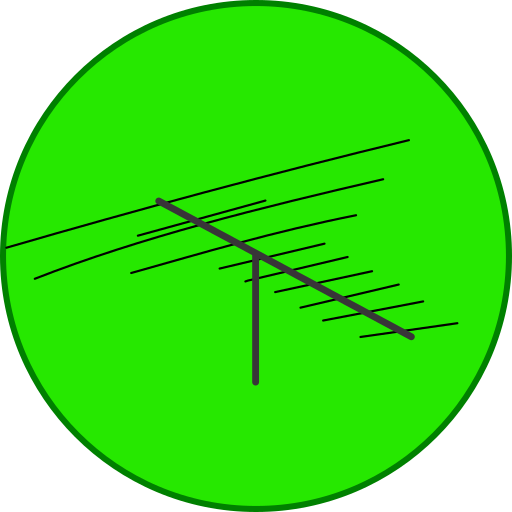4.3★

Android - iOS

### Description

Tools:
Q code - A list of common Q codes
92 Code - A list of common 92 codes
RST code - A list of RST codes
Distance calculator - Find the distance between two Maidenhead squares
Unit converter - Convert between units
Dipole antenna - Calculate the optimal lengths of a dipole antenna for a given frequency
Vertical antenna - Calculate the optimal lengths of a vertical antenna for a given frequency
Cubical quad antenna - Calculate the optimal lengths of a cubical quad antenna for a given frequency
Inverted Vee antenna - Calculate the optimal lengths of an inverted vee antenna for a given frequency
Ground plane antenna - Calculate the optimal lengths of a ground plane antenna for a given frequency
J pole antenna - Calculate the optimal lengths of a J pole antenna for a given frequency
VSWR - Convert between VSWR, Refection coefficient, return loss and mismatch loss
Three element Yagi antenna - Calculate the optimal lengths of a three-element Yagi antenna for a given frequency
Seven-element Yagi antenna - Calculate the optimal lengths of a seven element Yagi antenna for a given frequency
Wavelength frequency converter - Convert between wavelength and frequency
Air core inductor - Calculate the inductance or lengths of an air core inductor
Low pass filter - Calculate the units of an RC or RL LPF circuit
High pass filter - Calculate the units of an RC or RL HPF circuit
Ohm's law - Convert between voltage, current, resistance and power by entering two
Reactance - Calculate the reactance of a capacitor or inductor
Decibel calculator - Convert between decibel values or calculate dB by entering the power or voltage
Voltage divider - Calculate the output voltage or resistance of one resistor
Resistor Color codes - Find the resistance of a resistor by entering the colors of the bands
Resistors in parallel - Calculate the resistance of resistors in parallel
Resistors in series - Calculate the resistance of resistors in series
Capacitors in parallel - Calculate the capacitance of capacitors in parallel
Capacitors in series - Calculate the capacitance of capacitors in series
Coaxial cable - Calculate the properties of a coaxial cable
Morse code
CW abbreviations
NATO phonetic alphabet
You can upgrade to the Pro version to remove all ads and get the following features:
Half wave coax balun - Calculate the optimal length of a half wave coax balun for a given frequency
Narrowband balun - Calculate the inductance and capacitance of a narrowband balun
Small magnetic loop - Calculate the optimal lengths of a small magnetic loop for a given frequency
Full wave loop antenna - Calculate the optimal lengths of a full wave loop antenna for a given frequency
ERP and EIRP - Calculate and convert ERP and EIRP
RMS voltage - Calculate the RMS voltage
Pi attenuator - Calculate the values of the resistors by entering the attenuation and impedance
T attenuator - Calculate the values of the resistors by entering the attenuation and impedance
Inductors in series - Calculate the inductance of inductors in series
Inductors in parallel - Calculate the inductance of inductors in parallel
Doppler shift - Calculate the Doppler shift